# Sketch Constraints

Apply geometric relationships such as tangency and perpendicularity to sketch curves.

As you create geometry, many sketch constraints are automatically applied. For instance, if you draw a line that is nearly horizontal, a horizontal constraint will be created on that line automatically, making it precisely horizontal for you. When you modify a sketch, the constraints ensure that the intended relationships between the sketch entities remain intact.

## Apply or Remove Sketch Constraints

Apply sketch constraints to geometry to ensure that the intended relationships between sketch entities remain intact, or remove sketch constraints to allow more freedom to create free-form objects.

In sketch mode, click a sketch curve, and then select constraints or clear the check boxes.

Next to the Control Panel, a legend is displayed indicating by color which sketch curves are Underdefined, Fully Defined, Overconstrained, Fixed, and Imported.

Note: To hide constraints, right-click, and then deselect Show constraints.
Constraint Description
Fixed Lock the position of any sketch curve.

When a line is fixed, you can still move the end points to change the length. The end points of a line are separate entities and can be fixed independently.

Construction This is not a constraint.

Select this option to convert a sketch curve into construction geometry, which is used as a reference to line up or define other geometry.

Note: To hide construction geometry, right-click, and then deselect Show construction geometry.
HorizontalLines are forced to be horizontal.

Points are forced to have the same y (with respect to the sketch plane).

VerticalLines are forced to be horizontal.

Points are forced to have the same x (with respect to the sketch plane).

Parallel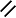Lines are forced to be parallel.
Perpendicular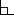Lines are forced to be perpendicular.
Tangent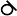Curves are forced to be tangent.
Coincident Geometry is forced to be coincident with another geometry.
Concentric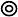Centers of arcs and circles are forced to be coincident.
Collinear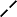Lines are forced to be coincident with the same straight line.
Equal Lines are forced to have the same length.

Arcs and circles are forced to have the same radius.

Midpoint A point is forced to be coincident with the midpoint of an open curve.
Symmetric Two curves are forced to be symmetric about a construction line (e.g., mirrored curves).